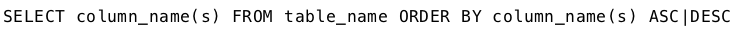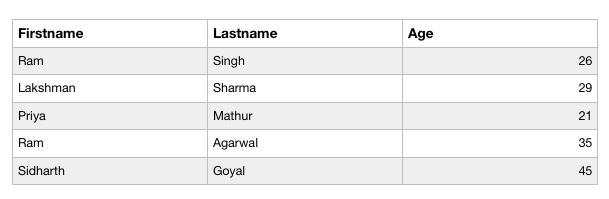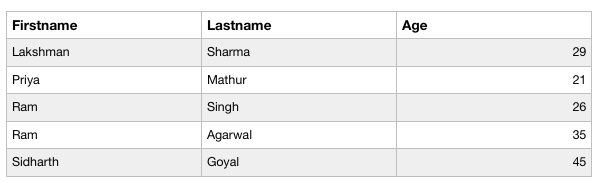# PHP | MySQL ORDER BY Clause

The ORDER BY Clause can be used along with the SELECT statement to sort the data of specific fields in an ordered way. It is used to sort the result-set in ascending or descending order.

Syntax :
The basic syntax of the Order By clause is –#### Implementation of the Order By Clause :

Let us consider the following table “Data” with three columns ‘FirstName’, ‘LastName’ and ‘Age’.To sort the FirstName column in the Data table in ascending order, the following code can be used.

Order By Clause using Procedural Method :

 ` 0){ ` `        ``echo "<``table``>"; ` `            ``echo "<``tr``>"; ` `            ``echo "<``th``>Firstname"; ` `            ``echo "<``th``>Lastname"; ` `            ``echo "<``th``>age"; ` `            ``echo ""; ` `        ``while(\$row = mysqli_fetch_array(\$res)){ ` `            ``echo "<``tr``>"; ` `                ``echo "<``td``>" . \$row['Firstname'] . ""; ` `                ``echo "<``td``>" . \$row['Lastname'] . ""; ` `                ``echo "<``td``>" . \$row['Age'] . ""; ` `            ``echo ""; ` `        ``} ` `        ``echo ""; ` `        ``mysqli_free_result(\$res); ` `    ``} else{ ` `        ``echo "No matching records are found."; ` `    ``} ` `} else{ ` `    ``echo "ERROR: Could not able to execute \$sql. " ` `                                ``. mysqli_error(\$link); ` `} ` `mysqli_close(\$link); ` `?> `

Output :Code Explanation :

1. The “res” variable stores the data that is returned by the function mysql_query().
2. Everytime mysqli_fetch_array() is invoked,it returns the next row from the res() set.
3. The while loop is used to loop through all the rows of the table “data”.

Order By Clause using Object Oriented Method :

 `connect_error); ` `} ` ` `  `\$sql = "SELECT * FROM Data ORDER BY Firstname"; ` `if(\$res = \$mysqli->query(\$sql)){ ` `    ``if(\$res->num_rows > 0){ ` `        ``echo "<``table``>"; ` `            ``echo "<``tr``>"; ` `                ``echo "<``th``>Firstname"; ` `                ``echo "<``th``>Lastname"; ` `                ``echo "<``th``>Age"; ` `            ``echo ""; ` `        ``while(\$row = \$res->fetch_array()){ ` `            ``echo "<``tr``>"; ` `                ``echo "<``td``>" . \$row['Firstname'] . ""; ` `                ``echo "<``td``>" . \$row['Lastname'] . ""; ` `                ``echo "<``td``>" . \$row['Age'] . ""; ` `            ``echo ""; ` `        ``} ` `        ``echo ""; ` `        ``\$res->free(); ` `    ``} else{ ` `        ``echo "No matching records are found."; ` `    ``} ` `} else{ ` `    ``echo "ERROR: Could not able to execute \$sql. "  ` `                                    ``. \$mysqli->error; ` `} ` ` `  `\$mysqli->close(); ` `?> `

Output :Order By Clause using PDO Method :

 `setAttribute(PDO::ATTR_ERRMODE,  ` `                        ``PDO::ERRMODE_EXCEPTION); ` `} catch(PDOException \$e){ ` `    ``die("ERROR: Could not connect. "  ` `                            ``. \$e->getMessage()); ` `} ` `try{ ` `    ``\$sql = "SELECT * FROM Data ORDER BY Firstname"; ` `    ``\$res = \$pdo->query(\$sql); ` `    ``if(\$res->rowCount() > 0){ ` `        ``echo "<``table``>"; ` `            ``echo "<``tr``>"; ` `                ``echo "<``th``>Firstname"; ` `                ``echo "<``th``>Lastname"; ` `                ``echo "<``th``>Age"; ` `            ``echo ""; ` `        ``while(\$row = \$res->fetch()){ ` `            ``echo "<``tr``>"; ` `                ``echo "<``td``>" . \$row['Firstname'] . ""; ` `                ``echo "<``td``>" . \$row['Lastname'] . ""; ` `                ``echo "<``td``>" . \$row['Age'] . ""; ` `            ``echo ""; ` `        ``} ` `        ``echo ""; ` `        ``unset(\$res); ` `    ``} else{ ` `        ``echo "No matching records are found."; ` `    ``} ` `} catch(PDOException \$e){ ` `    ``die("ERROR: Could not able to execute \$sql. "  ` `                                    ``. \$e->getMessage()); ` `} ` ` `  `unset(\$pdo); ` `?> `

Output :My Personal Notes arrow_drop_upIf you like GeeksforGeeks and would like to contribute, you can also write an article using contribute.geeksforgeeks.org or mail your article to contribute@geeksforgeeks.org. See your article appearing on the GeeksforGeeks main page and help other Geeks.

Please Improve this article if you find anything incorrect by clicking on the "Improve Article" button below.

Article Tags :
Practice Tags :

Be the First to upvote.

Please write to us at contribute@geeksforgeeks.org to report any issue with the above content.